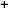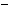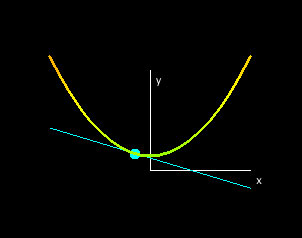Labware - MA35 Multivariable Calculus - Three Variable Calculus
 MA35 Labs 3 » Three Variable Calculus Contents3.2 Differentiation 3.2.1 Partial Derivatives 3.2.2 Critical Points 3.2.4 Chain Rule 3.2.5 Differentiability 3.4 Integration Search

Partial Derivatives

Text

The partial derivatives of a function of three variables are the slopes of the slice curves.Similarly the derivative of the x = x0, z = z0 slice curve f(x0,y,z0) with respect to y is denoted by fy(x0,y0,z0), called the first partial derivative of f with respect to y and the derivative of the x = x0, y = y0 slice curve f(x0,y0,z) with respect to z is denoted by fz(x0,y0,z0), called the first partial derivative of f with respect to z.Demos

 Partial DerivativesThis demo shows the slice curves at a point (P,f(P)), where P is a point in the domain. The slopes of the slice curves at P give the partial derivatives with respect to x, y and z at (P,f(P)). You can use the hotspot in the "Domain" window to get the partial derivatives of every point in the domain.Exercises

• 1. Position the hotspot at the origin. What are the slopes of the tangent lines at this point?
• 2. Where are the slopes of the tangent lines the greatest for all three slice curves?